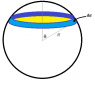# Magnetic moment of a solid, uniformly charged ball

• six7th
So now you have the area of the segment in terms of θ. Can you continue with the integration and show that it leads to the correct result?Integrating this over the whole sphere gives μ = \frac{ωq}{4πR^2} \int^{R}_{0} r^3 dr \int^{π}_{0} \sinθ dθ \int^{2π}_{0} d\phi μ = \frac{ωqR^2}{8} \int^{π}_{0} \sinθ dθ \int^{2π}_{0} d\phi μ = \frac{ωqR^2}{8} \cdot 2 \cdot

## Homework Statement

Show for a solid spherical ball of mass m rotating through its centre with a charge q uniformly distributed on the surface of the ball that the magnetic moment μ is related to the angular momentum L by the relation:

$\textbf{μ} = \frac{5q}{6mc}\textbf{L}$

## Homework Equations

$μ = IA$
$I = \frac{2}{5}MR^2$
$σ = \frac{q}{4πR^2}$
$\frac{1}{T} = \frac{ω}{2π}$

## The Attempt at a Solution

Split sphere into thin segments, each one has magnetic moment:

$dμ = dI \cdot dA$

where dA is the area enclosed by the segment:

$dA = 2π r dr$

The current dI on each segment is:

$dI = \frac{dq}{T} = \frac{ω}{2π} dq$

The charge on each segment is:

$dq = σ da$

Where da is the surface area of the segment:

$da = r^2\sinθ dθ d\phi$

This results in
$dq = σ \cdot r^2\sinθ dθ d\phi = \frac{q}{4πR^2} r^2\sinθ dθ d\phi$

We now have an expression for dI:

$dI = \frac{ωqr^2\sinθ}{8π^2R^2} dθ d\phi$

Now we can calculate the moment of each segment:

$dμ = \frac{ωqr^2\sinθ}{8π^2R^2} 2πr dr d\phi dθ$

Integrating this over the whole sphere gives

$μ = \frac{ωq}{4πR^2} \int^{R}_{0} r^3 dr \int^{π}_{0} \sinθ dθ \int^{2π}_{0} d\phi$

$μ = \frac{wqR^2}{8}$

Obviously this is not correct, the answer I should be getting is

$μ = \frac{wqR^2}{3}$

Where am I going wrong?

Last edited:
six7th said:
Split sphere into thin segments, each one has magnetic moment:

$dμ = dI \cdot dA$

where dA is the area enclosed by the segment:

$dA = 2π r dr$

Is that the correct expression for the area enclosed? Can you describe the area that you are talking about? Did you make a sketch?

For a normal loop of wire, A would be the area inside the loop so in this case dA is the area bounded by the surface, da, of the thin segment. I have tried using the volume of the segments but the equation for magnetic moments requires an area, so I couldn't see how this would work.

Edit: I have seen on similar problems that the radius of each segment is rsinθ making the area enclosed:

$A = πr^2\sin^2θ$

This may be where I am going wrong but I am struggling to see why the radius is rsinθ

Last edited:
The area enclosed by a circular strip on the sphere is shown in yellow in the figure. Can you express that area in terms of R and θ?

#### Attachments

•Area Spherical Strip.png
2.4 KB · Views: 619
I see it now, thank you!

The radius of the segment is just simple trigonometry,

$r = R\sinθ$

So the area now becomes:

$A = πR^2 \sin^2θ$

Good.

•1 person